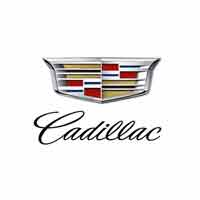# 凯迪拉克ATS-L•外观
•中控
•座椅
•细节
 本地参考价： 28.88 万元起 厂商指导价：28.88-42.88 万
 车市行情　｜　凯迪拉克ATS-L 降万
 最新年款： 2016款 级　别：中型车 结　构：4门5座三厢车 车身颜色： 红色 油　耗：6.8L/100km 排　量：2.0T 内饰配色： 红黑 保　修：3年或6万公里 变速箱：8挡手自一体|6挡手自一体

#### 20万元买什么汽车最符合自己 买好车为你支招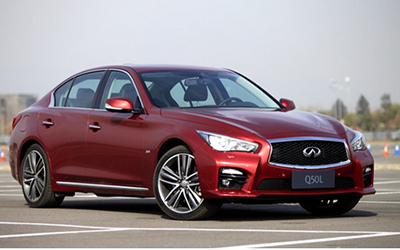#### 新款ATS-L正式上市 售价28.88-42.88万元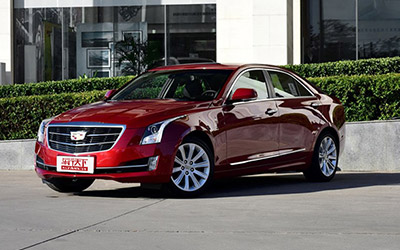#### 10月12日上市 凯迪拉克新款ATS-L官图曝光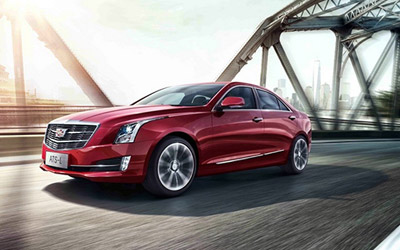2.0T 涡轮增压 279马力 国IV(国V) 官方指导价 本地参考价 车型信息 2014款 28T 精英型 D3运动版 6挡手自一体 前置后驱 35.38万暂无 参数　图片　＋对比 2016款 28T 技术型 8挡手自一体 前置后驱 29.88万暂无 参数　图片　＋对比 2016款 28T 时尚型 8挡手自一体 前置后驱 31.88万暂无 参数　图片　＋对比 2016款 28T 精英型 8挡手自一体 前置后驱 33.88万暂无 参数　图片　＋对比 2016款 28T 豪华型 8挡手自一体 前置后驱 36.88万暂无 参数　图片　＋对比 2016款 28T 领先型 8挡手自一体 前置后驱 42.88万暂无 参数　图片　＋对比 2014款 28T 精英型 6挡手自一体 前置后驱 33.88万暂无 参数　图片　＋对比 2014款 28T 豪华型 6挡手自一体 前置后驱 37.88万暂无 参数　图片　＋对比 2014款 28T 领先型 6挡手自一体 前置后驱 44.88万暂无 参数　图片　＋对比 2014款 28T 领先型 D3运动版 6挡手自一体 前置后驱 46.38万暂无 参数　图片　＋对比
 2.0T 涡轮增压 230马力 国IV(国V) 官方指导价 本地参考价 车型信息 2016款 25T 舒适型 6挡手自一体 前置后驱 28.88万暂无 参数　图片　＋对比 2016款 25T 时尚型 6挡手自一体 前置后驱 30.88万暂无 参数　图片　＋对比 2014款 25T 舒适型 6挡手自一体 前置后驱 28.88万暂无 参数　图片　＋对比 2014款 25T 时尚型 6挡手自一体 前置后驱 30.88万暂无 参数　图片　＋对比

### 绕车实拍 外观中控座椅细节全部### 凯迪拉克ATS-L 新闻资讯#### 20万元买什么汽车最符合自己 买好车为你支招

20万左右买什么车？往往千挑万选却越发眼花缭乱。今天，汽车电商买好车为你支招，让你尽享20万车型的轻奢主义。在买好车电商平台购车，不仅可...#### 新款ATS-L正式上市 售价28.88-42.88万元#### 10月12日上市 凯迪拉克新款ATS-L官图曝光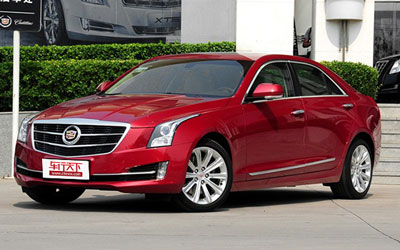#### 曝凯迪拉克ATS-L配置信息 8月15日上市#### 外观和动力受欢迎 数说凯迪拉克ATS-L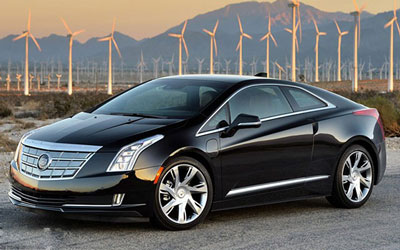#### 凯迪拉克新战略解析 品牌独立/更多新车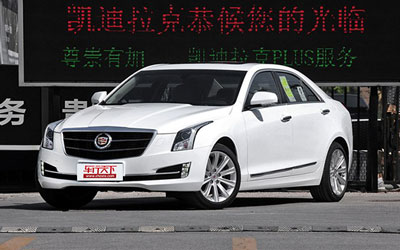#### 曝凯迪拉克ATS-L动力参数 两种动力配置#### 2015年上海通用推出车载4G LTE服务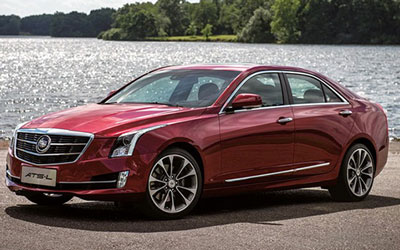### 猜你喜欢

﻿
• 快速找车
• 选择品牌
• 选择品牌
• A  奥迪
• A  阿斯顿·马丁
• A  阿尔法·罗密欧
• B  宝沃
• B  布加迪
• B  巴博斯
• B  保时捷
• B  宾利
• B  奔驰
• B  宝马
• B  本田
• B  别克
• B  标致
• B  比亚迪
• B  宝骏
• B  北汽制造
• B  北汽新能源
• B  北汽幻速
• B  北汽威旺
• B  北京汽车
• B  奔腾
• B  北汽绅宝
• B  北汽昌河
• C  长安欧尚
• C  长安
• C  长安凯程
• C  长城
• D  大众
• D  道奇
• D  DS
• D  东南
• D  东风风神
• D  东风风行
• D  东风小康
• D  东风风度
• D  东风
• F  福特
• F  丰田
• F  菲亚特
• F  法拉利
• F  福田
• F  福迪
• F  福汽启腾
• G  观致
• G  广汽传祺
• G  广汽吉奥
• G  GMC
• H  红旗
• H  汉腾汽车
• H  哈弗
• H  哈飞
• H  海格
• H  海马
• H  华颂
• H  黄海
• H  华泰
• H  恒天
• J  几何汽车
• J  捷达
• J  吉利汽车
• J  捷豹
• J  Jeep
• J  江淮
• J  江铃
• J  金杯
• J  九龙
• J  金旅
• K  凯翼
• K  凯迪拉克
• K  克莱斯勒
• K  科尼塞克
• K  卡威
• K  开瑞
• L  路虎
• L  林肯
• L  劳斯莱斯
• L  兰博基尼
• L  雷克萨斯
• L  铃木
• L  领克
• L  雷诺
• L  理念
• L  力帆
• L  莲花汽车
• L  猎豹
• L  路特斯
• L  陆风
• M  马自达
• M  MG
• M  MINI
• M  玛莎拉蒂
• M  摩根
• M  迈凯轮
• N  纳智捷
• O  欧拉
• O  欧宝
• O  讴歌
• O  欧朗
• Q  奇瑞
• Q  起亚
• Q  启辰
• R  日产
• R  荣威
• R  瑞麒
• S  SERES赛力斯
• S  三菱
• S  斯威汽车
• S  萨博
• S  smart
• S  斯柯达
• S  斯巴鲁
• S  思铭
• S  双龙
• S  上汽大通
• S  双环
• T  特斯拉
• T  腾势
• W  蔚来
• W  沃尔沃
• W  WEY
• W  五菱汽车
• W  五十铃
• W  威兹曼
• W  威麟
• X  现代
• X  雪佛兰
• X  星途
• X  雪铁龙
• X  小鹏汽车
• X  西雅特
• Y  一汽
• Y  英菲尼迪
• Y  英致
• Y  依维柯
• Y  野马汽车
• Y  永源
• Z  众泰
• Z  中华
• Z  中兴
• Z  知豆
• 选择车系
• 选择车系
• 车型对比
• 选择品牌
• 选择品牌
• A  奥迪
• A  阿斯顿·马丁
• A  阿尔法·罗密欧
• B  宝沃
• B  布加迪
• B  巴博斯
• B  保时捷
• B  宾利
• B  奔驰
• B  宝马
• B  本田
• B  别克
• B  标致
• B  比亚迪
• B  宝骏
• B  北汽制造
• B  北汽新能源
• B  北汽幻速
• B  北汽威旺
• B  北京汽车
• B  奔腾
• B  北汽绅宝
• B  北汽昌河
• C  长安欧尚
• C  长安
• C  长安凯程
• C  长城
• D  大众
• D  道奇
• D  DS
• D  东南
• D  东风风神
• D  东风风行
• D  东风小康
• D  东风风度
• D  东风
• F  福特
• F  丰田
• F  菲亚特
• F  法拉利
• F  福田
• F  福迪
• F  福汽启腾
• G  观致
• G  广汽传祺
• G  广汽吉奥
• G  GMC
• H  红旗
• H  汉腾汽车
• H  哈弗
• H  哈飞
• H  海格
• H  海马
• H  华颂
• H  黄海
• H  华泰
• H  恒天
• J  几何汽车
• J  捷达
• J  吉利汽车
• J  捷豹
• J  Jeep
• J  江淮
• J  江铃
• J  金杯
• J  九龙
• J  金旅
• K  凯翼
• K  凯迪拉克
• K  克莱斯勒
• K  科尼塞克
• K  卡威
• K  开瑞
• L  路虎
• L  林肯
• L  劳斯莱斯
• L  兰博基尼
• L  雷克萨斯
• L  铃木
• L  领克
• L  雷诺
• L  理念
• L  力帆
• L  莲花汽车
• L  猎豹
• L  路特斯
• L  陆风
• M  马自达
• M  MG
• M  MINI
• M  玛莎拉蒂
• M  摩根
• M  迈凯轮
• N  纳智捷
• O  欧拉
• O  欧宝
• O  讴歌
• O  欧朗
• Q  奇瑞
• Q  起亚
• Q  启辰
• R  日产
• R  荣威
• R  瑞麒
• S  SERES赛力斯
• S  三菱
• S  斯威汽车
• S  萨博
• S  smart
• S  斯柯达
• S  斯巴鲁
• S  思铭
• S  双龙
• S  上汽大通
• S  双环
• T  特斯拉
• T  腾势
• W  蔚来
• W  沃尔沃
• W  WEY
• W  五菱汽车
• W  五十铃
• W  威兹曼
• W  威麟
• X  现代
• X  雪佛兰
• X  星途
• X  雪铁龙
• X  小鹏汽车
• X  西雅特
• Y  一汽
• Y  英菲尼迪
• Y  英致
• Y  依维柯
• Y  野马汽车
• Y  永源
• Z  众泰
• Z  中华
• Z  中兴
• Z  知豆
• 选择车系
• 选择车系
• 选择车型
• 选择车型
• 意见反馈